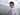# 8. 字符串转换整数 (atoi)

November 24, 2019

https://leetcode-cn.com/problems/string-to-integer-atoi/

``````func myAtoi(str string) int {
MIN := -2147483648
MAX := 2147483647
isNegative := false
hasNum := false
var res int
for _, c := range str {
if res == 0 && !hasNum {
// 32 - space，0 - 空字符，10 - 换行
if c == 32 || c == 0 || c == 10 {
continue
}
// 43 +，45 -
if c == 45 {
isNegative = true
hasNum = true
continue
} else if c == 43 {
hasNum = true
continue
}
}
// 0~9 - 48~57
if c < 48 || c > 57 {
break
}
if isNegative {
if -res < (MIN + int(c) - 48) / 10 {
return MIN
}
} else if res > (MAX - int(c) + 48) / 10 {
return MAX
}
res = res * 10 + int(c) - 48
hasNum = true
}

if isNegative {
res = -res
}

return res
}``````